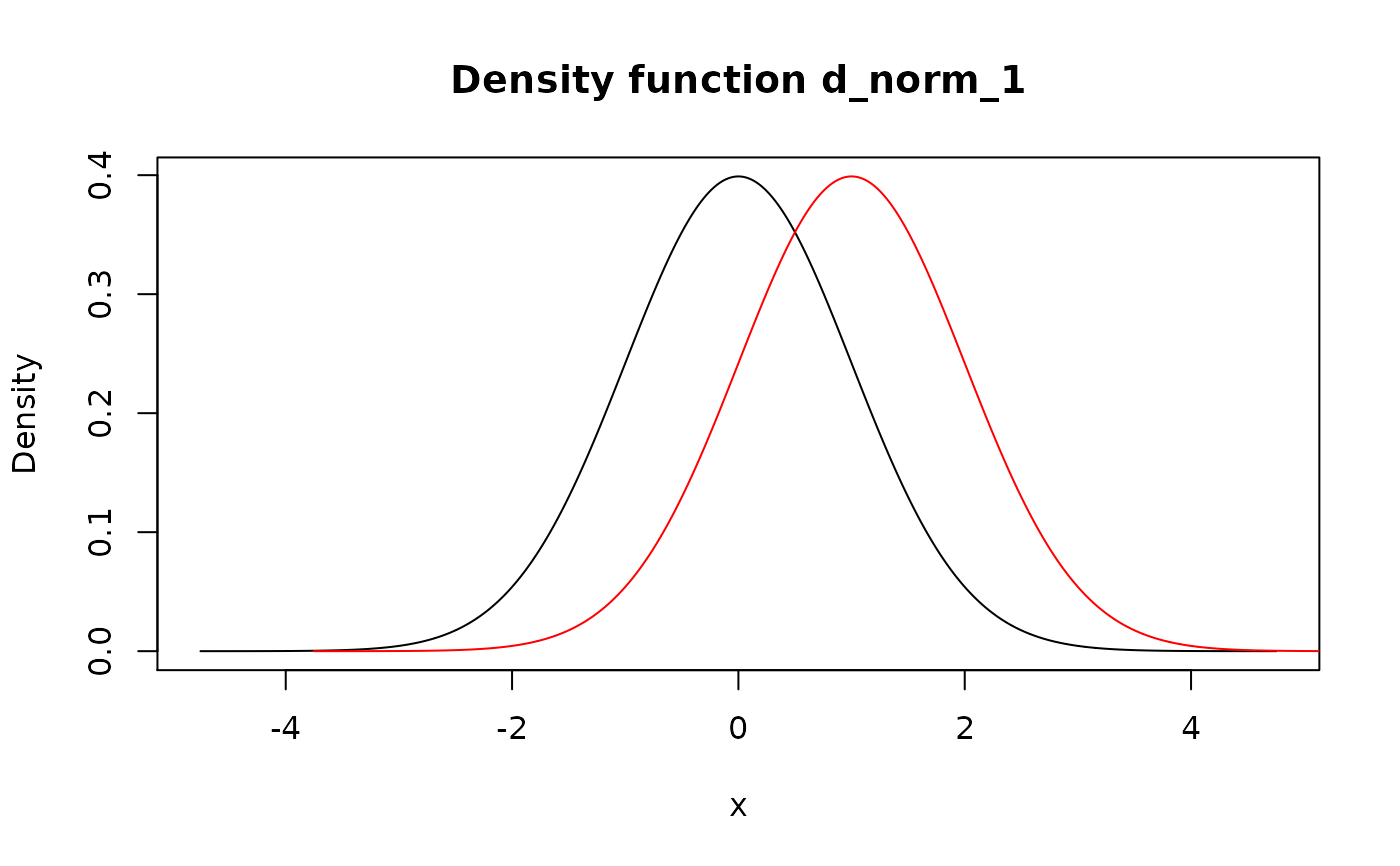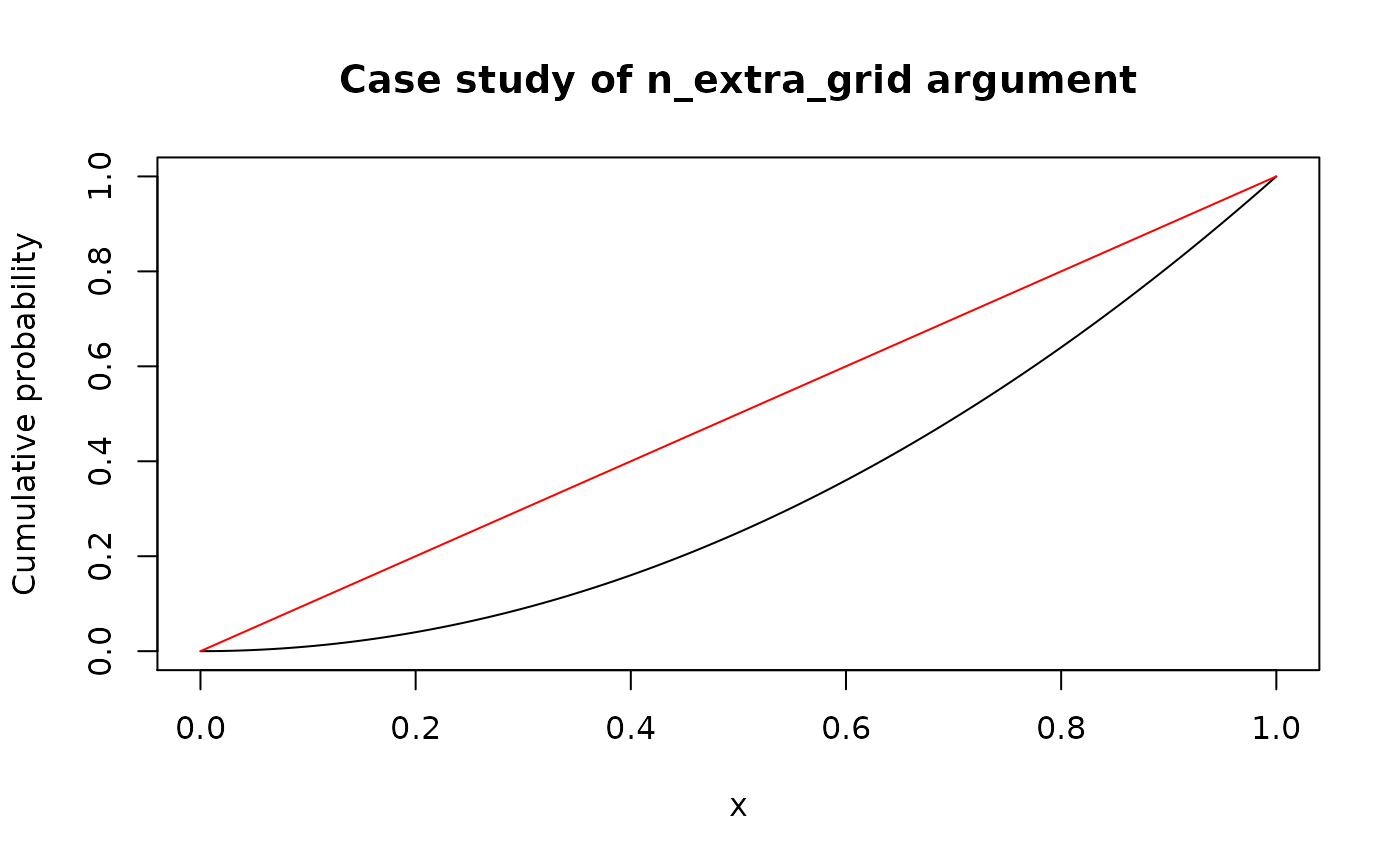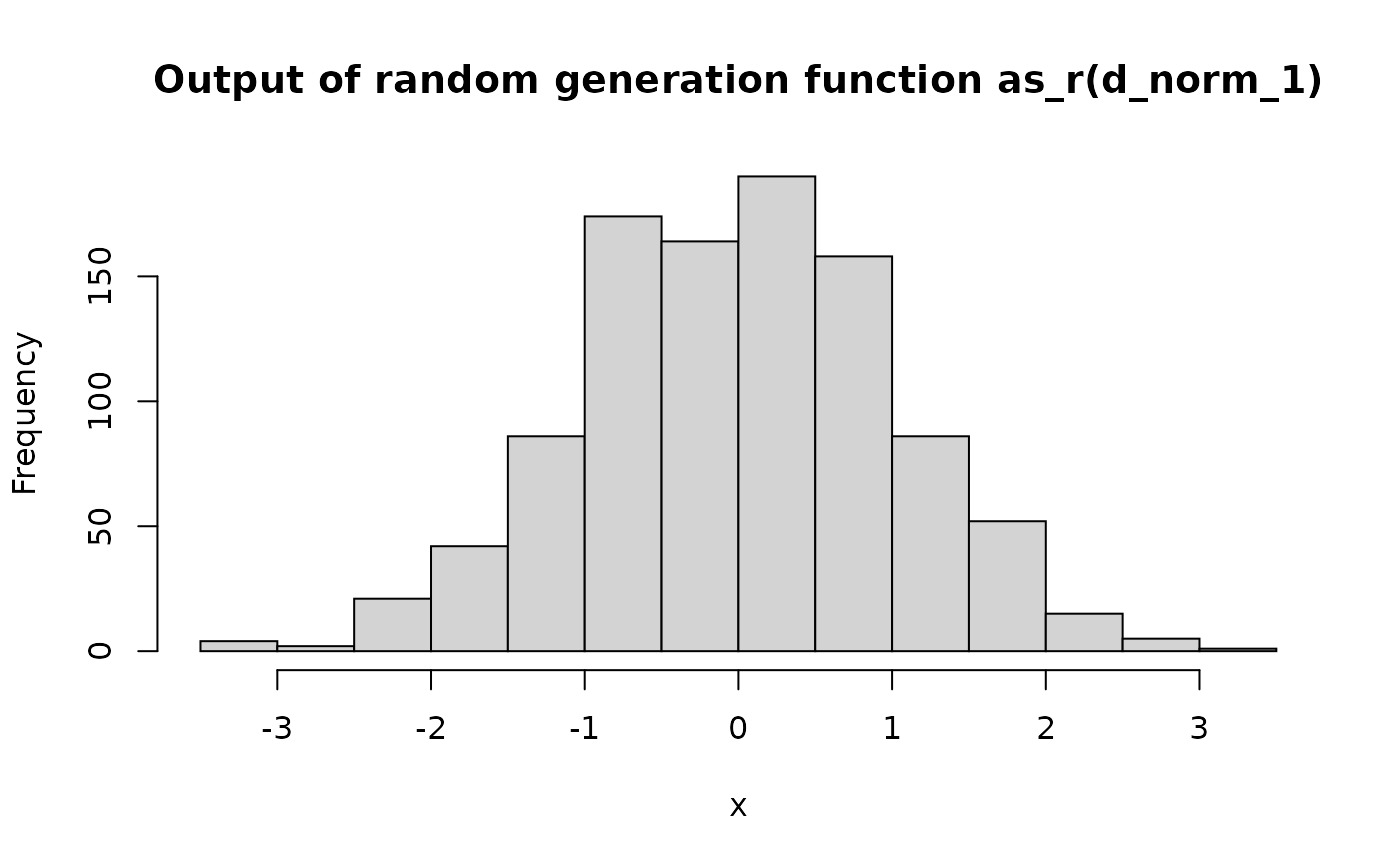Pdqr-functions have their own methods for plot() and lines() (except r-functions, see Details).

# S3 method for p
plot(x, y = NULL, n_extra_grid = 1001, ...)

# S3 method for d
plot(x, y = NULL, n_extra_grid = 1001, ...)

# S3 method for q
plot(x, y = NULL, n_extra_grid = 1001, ...)

# S3 method for r
plot(x, y = NULL, n_sample = 1000, ...)

# S3 method for p
lines(x, n_extra_grid = 1001, ...)

# S3 method for d
lines(x, n_extra_grid = 1001, ...)

# S3 method for q
lines(x, n_extra_grid = 1001, ...)

## Arguments

x Pdqr-function to plot. Argument for compatibility with plot() signature. Doesn't used. Number of extra grid points at which to evaluate pdqr-function (see Details). Supply NULL or 0 to not use extra grid. Other arguments for plot() or hist() (in case of plotting r-function). Size of a sample to be generated for plotting histogram in case of an r-function.

## Value

Output of invisible() without arguments, i.e. NULL without printing.

## Details

Main idea of plotting pdqr-functions is to use plotting mechanisms for appropriate numerical data.

Plotting of type discrete functions:

• P-functions are plotted as step-line with jumps at points of "x" column of "x_tbl" metadata.

• D-functions are plotted with vertical lines at points of "x" column of "x_tbl" with height equal to values from "prob" column.

• Q-functions are plotted as step-line with jumps at points of "cumprob" column of "x_tbl".

• R-functions are plotted by generating sample of size n_sample and calling hist() function.

Plotting of type continuous functions:

• P-functions are plotted in piecewise-linear fashion at their values on compound grid: sorted union of "x" column from "x_tbl" metadata and sequence of length n_extra_grid consisting from equidistant points between edges of support. Here extra grid is needed to show curvature of lines between "x" points from "x_tbl" (see Examples).

• D-functions are plotted in the same way as p-functions.

• Q-functions are plotted similarly as p- and d-functions but grid consists from union of "cumprob" column of "x_tbl" metadata and equidistant grid of length n_extra_grid from 0 to 1.

• R-functions are plotted the same way as type "discrete" ones: as histogram of generated sample of size n_sample.

Other pdqr methods for generic functions: methods-group-generic, methods-print

## Examples

d_norm_1 <- as_d(dnorm)
d_norm_2 <- as_d(dnorm, mean = 1)

plot(d_norm_1)lines(d_norm_2, col = "red")# Usage of n_extra_grid is important in case of "continuous" p- and
# q-functions
simple_p <- new_p(data.frame(x = c(0, 1), y = c(0, 1)), "continuous")
plot(simple_p, main = "Case study of n_extra_grid argument")lines(simple_p, n_extra_grid = 0, col = "red")# R-functions are plotted with histogram
plot(as_r(d_norm_1))